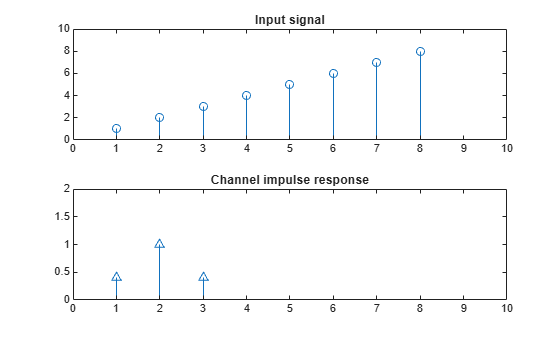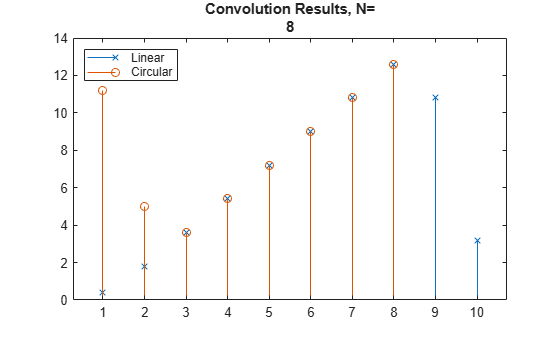# Equalization, Convolution, and Cyclic Prefix Addition

This example introduces frequency domain equalization and shows how to convert circular convolution to linear convolution. When considering a linear channel model, the received signal is the convolution of the transmitted signal with the channel impulse response. In the frequency domain, the received signal $\mathit{Y}\left(\mathit{f}\right)$ is the linear convolution of the transmitted signal $\mathit{U}\left(\mathit{f}\right)$ with the channel impulse response $\mathit{H}\left(\mathit{f}\right)$:

`$\mathit{Y}\left(\mathit{f}\right)=\mathit{H}\left(\mathit{f}\right)\cdot \mathit{U}\left(\mathit{f}\right)$`

OFDM receivers use frequency domain equalization to recover the original transmitted signal, so that:

`$\mathit{U}\left(\mathit{f}\right)=\frac{\mathit{Y}\left(\mathit{f}\right)}{\mathit{H}\left(\mathit{f}\right)}$`

FFT processing yields the circular convolution of u with h. For the circular convolution of u and h to be equivalent to the linear convolution, u and h must be padded with zeros to a length of at least `(length(u) + length(h) - 1)` before you take the discreet Fourier transform (DFT). After you invert the product of the DFTs, retain only the first N + L - 1 elements. For an example that demonstrates this process, see the Linear and Circular Convolution topic.

Define a short input signal, u`1`, and channel impulse response, `h`. The input signal must be longer than the channel impulse response. Display a stem plot of the signals.

```u1 = 1:8; h = [0.4 1 0.4]; figure subplot(2,1,1) stem(u1); axis([0 10 0 10]) title("Input signal") subplot(2,1,2) stem(h); axis([0 10 0 2]) title("Channel impulse response")```Compare the circular and linear convolution of `u1` with `h`. Perform linear and circular convolution by using the `conv` and `cconv` functions, respectively. The smearing effects due to the nonideal channel cause the linear and circular convolution to yield different results at some points. A cyclic prefix (CP) enables effective use of OFDM in a nonideal channel with unknown propagation delay.

```N = length(u1); yl1 = conv(u1,h); yc1 = cconv(u1,h,N); figure; stem(yl1,"x") hold on; stem(yc1,"o") title(["Convolution Results - N=",int2str(N)]) legend ("Linear","Circular","Location","northwest")```For OFDM processing, the necessary padding for the circular convolution is provided by adding a CP rather than zero-padding the signals. Adding a CP that repeats the end samples of the symbol enables:

• Modeling of the linear convolution of a frequency-selective multipath channel as circular convolution

• Use of an FFT to compute the convolution

• Simple frequency domain processing for channel estimation, equalization, and synchronization

• Repeated samples to be used in forward error correction schemes

```L = length(h); % Length of channel N = length(u1); % Length of input signal ucp = u1(N-L+1:N); % Use last samples of input signal as the CP u2 = [ucp u1]; % Prepend the CP to the input signal yl2 = conv(u2,h); % Convolution of input+CP and channel yl2 = yl2(L+1:end); % Remove CP to compare signals figure; stem(yc1,"x") hold on; stem(yl2,"o") title("Convolution Results with Cyclic Prefix") legend ("Linear","Circular","Location","northwest")```Compare the linear and circular convolution sequences.

```if max(yc1 - yl2(1:N)) < 1e-8 disp("Linear and circular convolution sequences match.") else disp("Received symbols do not match transmitted symbols.") end```
```Linear and circular convolution sequences match. ```